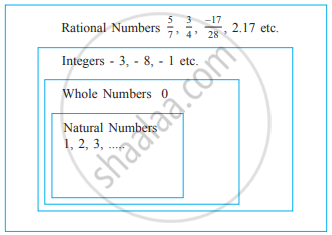# Rational Numbers

#### definition

Rational Number: A number that can be expressed in the form p/q, where p and q are integers and q ≠ 0, is called a rational number. The numbers -2/7, 3/8, 3 etc. are rational numbers.

# Rational Numbers:

• The word rational arises from the term ratio.

• A number that can be expressed in the form "p"/"q", where p and q are integers and q ≠ 0, is called a rational number.

• In "p"/"q", the integer p is the numerator, and the integer q (q ≠ 0) is the denominator.

• Thus, in (-3)/7, the numerator is - 3 and the denominator is 7.

• All Fractional numbers are rational numbers. For example, 3/7, 5/8, 0/9, 7 1/4, 29/4 etc. are rational numbers.

• All decimal numbers are rational numbers.

0.987 = 0.987 xx (1000)/(1000) = (0.987 xx 1000)/(1000) = (987)/(1000).

• All integers are rational numbers. For example, The integer - 5 is a rational number, because you can write it as (- 5)/1.
• The integer 0 can also be written as 0 = 0/2 or 0/7 etc. Hence, it is also a rational number.## Need for Rational Numbers:

• We can represent a distance of 750 m above sea level as 3/4 km. But Can we denote the distance of 3/4 km below sea level by - 3/4?
• We can see -3/4is neither an integer nor a fractional number. We need to extend our number system to include such numbers. So, we need rational numbers.
If you would like to contribute notes or other learning material, please submit them using the button below.

### Shaalaa.com

What are Rational Numbers? [00:07:30]
S
0%